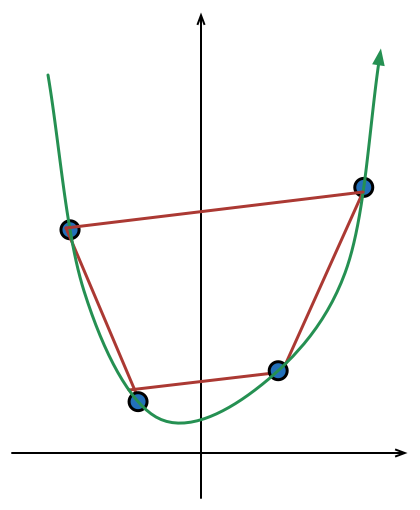Geometry Level 3Consider a curve $y=ax^2 +bx+c$ with $a, b, c \in \mathbb{N}$ which passes through four points $A(-2,3),B(-1,1),C(\alpha ,\beta ),D(2,7)$.

All these points are taken in given order for constructing a convex quadrilateral, which has maximum possible area.

Find minimum possible value of $a+b+c+2\alpha +4\beta.$

×